Heat Transfer in Boiling & Condensation - 3

# Heat Transfer in Boiling & Condensation - 3 Notes | Study Heat Transfer - Mechanical Engineering

## Document Description: Heat Transfer in Boiling & Condensation - 3 for Mechanical Engineering 2022 is part of Boiling & Condensation for Heat Transfer preparation. The notes and questions for Heat Transfer in Boiling & Condensation - 3 have been prepared according to the Mechanical Engineering exam syllabus. Information about Heat Transfer in Boiling & Condensation - 3 covers topics like and Heat Transfer in Boiling & Condensation - 3 Example, for Mechanical Engineering 2022 Exam. Find important definitions, questions, notes, meanings, examples, exercises and tests below for Heat Transfer in Boiling & Condensation - 3.

Introduction of Heat Transfer in Boiling & Condensation - 3 in English is available as part of our Heat Transfer for Mechanical Engineering & Heat Transfer in Boiling & Condensation - 3 in Hindi for Heat Transfer course. Download more important topics related with Boiling & Condensation, notes, lectures and mock test series for Mechanical Engineering Exam by signing up for free. Mechanical Engineering: Heat Transfer in Boiling & Condensation - 3 Notes | Study Heat Transfer - Mechanical Engineering
 1 Crore+ students have signed up on EduRev. Have you?

6.4 Film condensation on a vertical flat plate

Figure 6.4 shows a vertical wall very long in z-direction. The wall is exposed to a condensable vapour. The condensate film is assumed to be fully developed laminar flow with zero interfacial shear and constant liquid properties. It is also assumed that the vapour is saturated and the heat transfer through the condensate film occurs by condensation only and the temperature profile is assumed to be linear.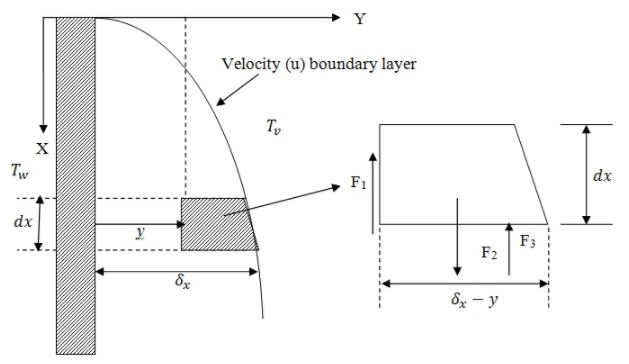Fig. 6.4: Condensation of film in laminar flow

The wall temperature is maintained at temperature Tw and the vapour temperature at the edge of the film is the saturation temperature Tv. The condensate film thickness is represented by δx, a function of x. A fluid element of thickness dx was assumed with a unit width in the z-direction.
The force balance on the element provides,

F1 = F2 - F3

where, shear force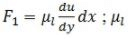is the viscosity of the condensate (liquid). In the subsequent sections of this module, the subscripts l and v will represent liquid and vapour phase.

Gravity force, F2 = ρlg (δx - y)dx; and

Buoyancy force, F3 = ρvg (δx - y)dx

Thus,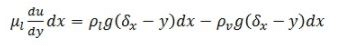On integrating for the following boundary condition, u = 0 at y = 0; no slip condition.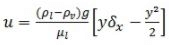(6.6)

Equation 6.6 shows the velocity profile in the condensate falling film.

The corresponding mass flow rate of the condensate for dy thickness and unit width of the film,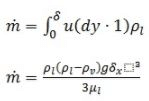(6.7)

where dy is the length of the volume element at y distance. The rate of condensation for  dx.1 (over element surface) area exposed to the vapour can be obtained from the rate of heat transfer through this area.

The rate of heat transfer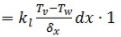The thermal conductivity of the liquid is represented by kl. The above rate of heat transfer is due to the latent heat of condensation of the vapour. Thus,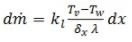(6.8)

The specific latent heat of condensation is represented by λ. On solving eqs.6.7 and 6.8, for boundary layer conditions (x = 0; δx = 0)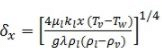(6.9)

The eq. 6.9 gives the local condensate film thickness at any location x. If h is the film heat transfer coefficient for the condensate film, heat flux through the film at any location  is,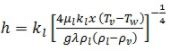(6.10 a)

The local Nusselt number will be,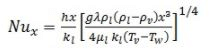We can also calculate the average heat transfer coefficient along the length of the surface,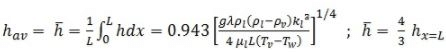(6.10 b)

In eq. 6.10, the liquid properties can be taken at the mean film temperature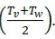The equation 6.10 is applicable for Pr > 0.5 and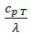≤ 1.0

It can also be understood that at any location on the plate the liquid film temperature changes from Tv to Tw. It indicates that apart from latent heat some amount of sensible heat will also be removed. Thus, to take this into account and to further improve the accuracy of Nusselt’s equation (eq. 6.10), a modified latent heat term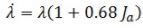can be used in place of λ. The term Ja is called the Jacob number as is defined by eq. 6.11. All the properties are to be evaluated at film temperature.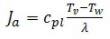(6.11)

In the previous discussion we have not discussed about the ripples or turbulent condition of the condensate film as it grows while coming down from the vertical wall. The previous discussion was applicable only when the flow in the condensate film was 1-D and the velocity profile was half parabolic all along the length of the wall. However, if the rate of condensation is high or the height of the condensing wall is more, the thickness of the condensate film neither remains small nor the flow remains laminar.

The nature of the flow is determined by the film Reynolds number (Ref). The local average liquid velocity in the film can be obtained by eq. 6.6.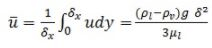Now, the Ref can be calculated by,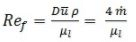(6.12)

where D is the hydraulic diameter of the condensate film. The hydraulic diameter can be calculated by the flow area (δx.1) and wetted perimeter (unit breadth, thus 1). It has been found that, if

Case 1: Ref ≤ 30; the film remains laminar and the free surface of the film remains wave free.
Case 2:  30 < Ref < 1600; the film remains laminar but the waves and ripples appear on the surface.
Case 3:  Ref ≥ 1600; the film becomes turbulent and surface becomes wavy.

The corresponding average heat transfer coefficient can be calculated by the following correlation,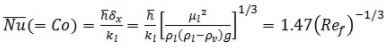:  for Case 1

(It is same as eq. 6.10 if Ref is taken at the bottom of the wall.)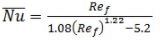:  for Case 2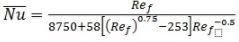:  for Case 3

The Nusselt number in case-1 is defined as Modified Nusselt number or condensation number (Co).

The above relations may also be used for condensation inside or outside of a vertical tube if the tube diameter is very large in comparison to condensate film thickness. Moreover, the relations are valid for the tilted surfaces also. If the surface make an angle “θ” from the vertical plane the “g” will be replaced by “g.cosθ” in the above equations

The document Heat Transfer in Boiling & Condensation - 3 Notes | Study Heat Transfer - Mechanical Engineering is a part of the Mechanical Engineering Course Heat Transfer.
All you need of Mechanical Engineering at this link: Mechanical Engineering

## Heat Transfer

58 videos|70 docs|85 tests
 Use Code STAYHOME200 and get INR 200 additional OFF

## Heat Transfer

58 videos|70 docs|85 tests

Track your progress, build streaks, highlight & save important lessons and more!

,

,

,

,

,

,

,

,

,

,

,

,

,

,

,

,

,

,

,

,

,

;